# Algebra

## Activities

Algebra In Action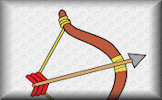Real life problems adapted from an old Mathematics textbook which can be solved using algebra.

Algebra Pairs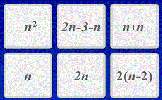The classic Pelmanism or pairs game requiring you to match equivalent expressions.

Algebraic Fractions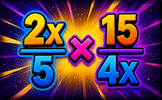A mixture of algebraic fraction calculations and simplifications.

Algebraic Notation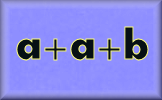Simplification using the normal conventions of algebra.

Algebraic Perimeters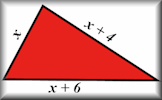Questions about the perimeters and areas of polygons given as algebraic expressions.

BIDMAS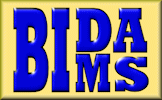A self marking exercise testing the application of BIDMAS, an acronym describing the order of operations used when evaluating expressions.

BIDMAS Game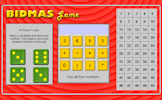An online interactive game celebrating the order of mathematical operations.

Binomial Theorem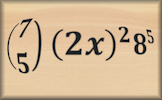Exercises in the process of expanding powers of binomial expressions and finding specific coefficients.

Brackets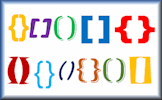Expand algebraic expressions containing brackets and simplify the resulting expression in this self marking exercise.

Changing The Subject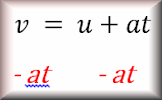Rearrange a formula in order to find a new subject in this self marking exercise.

Clouds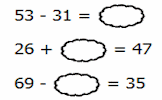Can you work out which numbers are hidden behind the clouds in these calculations?

Collecting Like Terms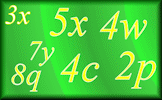Practise your algebraic simplification skills with this self marking exercise.

Completing the Square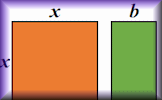Practise this technique for use in solving quadratic equations and analysing graphs.

Connecting Rules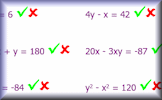If you are given the values of x and y which of these equations is correct?

Equations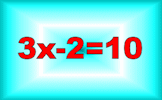A series of exercises, in increasing order of difficulty, requiring you to solve linear equations. The exercises are self marking.

Factorising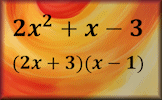Practise the skills of algebraic factorisation in this structured online self marking exercise.

Formulae to Remember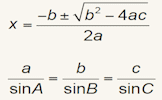The traditional pairs or pelmanism game adapted to test recognition for formulae required to be memorised for GCSE exams.

Function Builder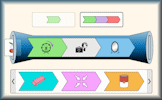An interactive function machine for patterns, numbers and equations.

Functions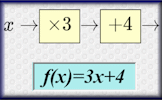An online exercise on function notation, inverse functions and composite functions.

Graph Equation PairsMatch the equation with its graph. Includes quadratics, cubics, reciprocals, exponential and the sine function.

Identity, Equation or Formula?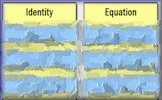Arrange the given statements in groups to show whether they are identities, equations or formulae.

Inequalities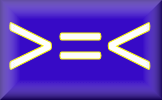Check that you know what inequality signs mean and how they are used to compare two quantities. Includes negative numbers, decimals, fractions and metric measures.

Iteration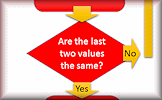Find approximate solutions to equations numerically using iteration.

Lemon LawChange the numbers on the apples so that the number on the lemon is the given total.

Linear Programming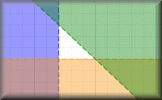A selection of linear programming questions with an interactive graph plotting tool.

Matchstick Patterns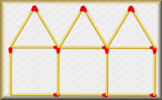Create a formula to describe the nth term of a sequence by examining the structure of the diagrams.

Missing Lengths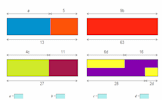Find the unknown lengths in the given diagrams and learn some algebra at the same time.

Nevertheless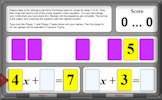Players decide where to place the cards to make an equation with the largest possible solution.

Old Equations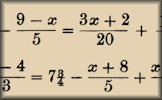Solve these linear equations that appeared in a book called A Graduated Series of Exercises in Elementary Algebra by Rev George Farncomb Wright published in 1857.

Online Psychic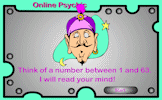Let the psychic read the cards and magically reveal the number you have secretly chosen. What is the mathematics that makes this trick work?

Pascal's Triangle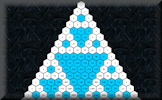Get to know this famous number pattern with some revealing learning activities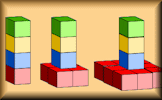Deduce expressions to calculate the nth term of quadratic and cubic sequences.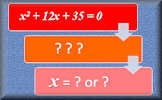Solve these quadratic equations algebraically in this seven-level, self-marking online exercise.

Recurring Decimals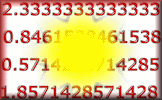Change recurring decimals into their corresponding fractions and vica versa.

Simultaneous Solutions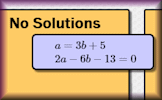Arrange the given pairs of simultaneous equations in groups to show whether they have no solution, one solution or infinite solutions.

Stable Scales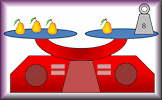Ten balance puzzles to prepare you for solving equations.

Substitution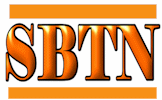Substitute the given values into the algebraic expressions.

Superfluous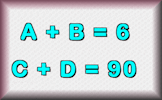Find a strategy to figure out the values of the letters used in these calculations.

Think of a NumberTen students think of a number then perform various operations on that number. You have to find what the original numbers were.

Words and Concepts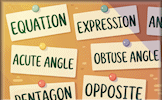Fill in the missing words to show an understanding of the vocabulary of equations, inequalities, terms and factors.

Writing Expressions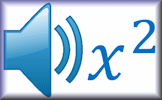Listen to the voice saying the algebraic expression then write it in its simplest form.

## Investigations

Calendar Maths Investigation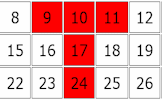Investigate the connection between the numbers in a T shape drawn on this month's calendar.

Crossing the River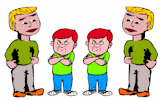Two men and two boys want to cross a river and they only have one canoe which will only hold one man or two boys.

Function BuilderAn interactive function machine for patterns, numbers and equations.

Lamp Posts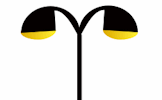What is the greatest number of lamp posts that would be needed for a strange village with only straight roads?

Steps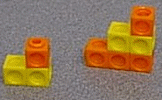Investigate the numbers associated with this growing sequence of steps made from Multilink cubes.

## Videos

This is the main Transum help video on Algebra.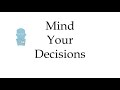A computationally-efficient, natural, and easy-to-remember algorithm for solving general quadratic equations.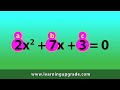A song from Math Upgrade dot com.

Simultaneous Equations (Elimination)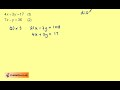This video demonstrates how to solve simultaneous equations by elimination.

Simultaneous Equations (Substitution)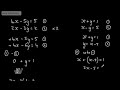This video demonstrates how to solve simultaneous equations by substitution.

## Visual Aids

eQuation Generator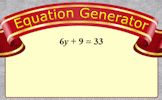An unlimited supply of linear equations just waiting to be solved. Project for the whole class to see then insert the working in your own style.

How old was Diophantus?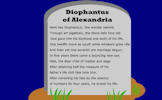An ancient riddle which can be answered by solving an equation containing fractions.

Online PsychicLet the psychic read the cards and magically reveal the number you have secretly chosen. What is the mathematics that makes this trick work?

Substitution Examples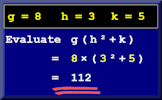A projectable set of animated examples to help prepare pupils to do the Substitution online exercise.

## Starters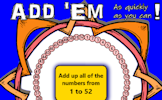Add up a sequence of consecutive numbers. Can you find a quick way to do it?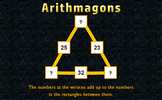Arithmagons
This lesson starter requires pupils to find the missing numbers in this partly completed arithmagon puzzle.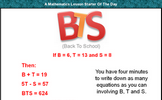BTS
You have four minutes to write down as many equations as you can involving B, T and S.Cars
Calculate the total cost of four cars from the information given.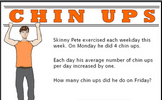Chin-Ups
Work out the number of chin ups the characters do on the last day of the week give information about averages.Christmas Presents
Work out the total cost of five Christmas presents from the information given.Connecting Rules
Give 20 rules connecting x and y given their values.Giraffe
The height of this giraffe is three and a half metres plus half of its height. How tall is the giraffe?Half Hearted
Find the number which when added to the top (numerator) and bottom (denominator) of each fraction make it equivalent to one half.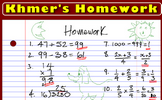Khmer's Homework
Check a student's homework. If you find any of the answers are wrong write down a sentence or two explaining what he did wrong.Know WeighLemon Law
Change the numbers on the apples so that the number on the lemon is the given total.Less Than
This mathematics lesson starter invites pupils to interpret a three part algebraic inequality.Light Shopping
A lamp and a bulb together cost 32 pounds. The lamp costs 30 pounds more than the bulb. How much does the bulb cost?Lost Sheep
Which algebraic expression is the odd one out?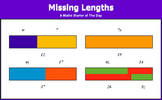Missing Lengths
Introduce linear equations by solving these problems about lengths.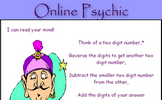Mystic Maths
Work out why subtracting a two digit number from its reverse gives a multiple of nine.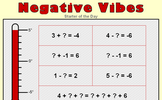Negative Vibes
Practise techniques for answering questions involving negative numbers.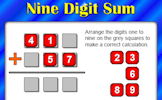Planet Numpair
The sum and product are given, can you find the two numbers?PYA
You have four minutes to write down as many equations as you can involving the given letters.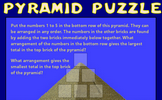Pyramid Puzzle
Arrange numbers at the bottom of the pyramid which will give the largest total at the top.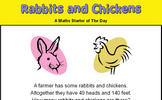Rabbits and Chickens
There are some rabbits and chickens in a field. Calculate how many of each given the number of heads and feet.Rail Weigh
Record the weights of the trains to work out the weight of a locomotive and a coach. A real situation which produces simultaneous equations.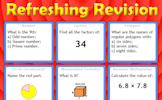Refreshing Revision
It is called Refreshing Revision because every time you refresh the page you get different revision questions.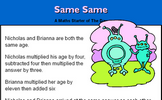Same Same
A problem involving two people's ages which can be solved using algebra.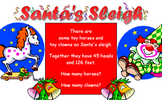Santa's Sleigh
Work out the number of clowns and horses given the number of heads and feet.Sea Shells
A question which can be best answered by using algebra.Simultaneous Occasions
A problem which can best be solved as a pair of simultaneous equations.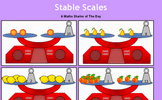Stable Scales
Solve these balance puzzles by taking the same away from both sides. An introduction to linear equations.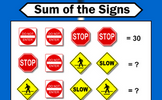Sum of the Signs
Each traffic sign stands for a number. Some of the sums of rows and columns are shown. What numbers might the signs stand for?Summer Holidays
How many children and how many donkeys are on the beach? You can work it out from the number of heads and the number of feet!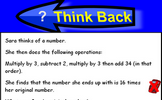Think Back
A problem which can be answered by forming an algebraic equation then solving it.THOAN
THOAN stands for 'Think of a number' and there are four randomly generated THOAN puzzles to solve.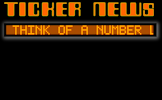Ticker News
A Think Of A Number problem presented as a news ticker.

84 items are currently in this category.

Teachers might find the complete Algebra Topic List useful.

Pupils begin their study of algebra by investigating number patterns. Later they construct and express in symbolic form and use simple formulae involving one or many operations. They use brackets, indices and other constructs to apply algebra to real word problems. This leads to using algebra as an invaluable tool for solving problems, modelling situations and investigating ideas.

If this topic were split into four sub topics they might be:

Creating and simplifying expressions;
Expanding and factorising expressions;
Substituting and using formulae;
Solving equations and real life problems;

This is a powerful topic and has strong links to other branches of mathematics such as number, geometry and statistics. See also "Number Patterns", "Negative Numbers" and "Simultaneous Equations".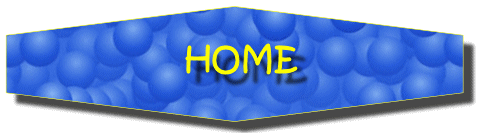For All: